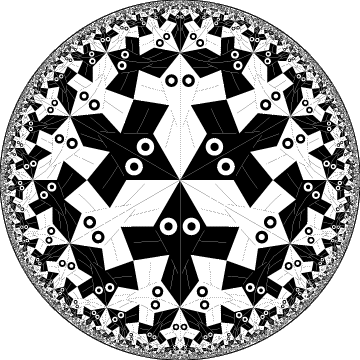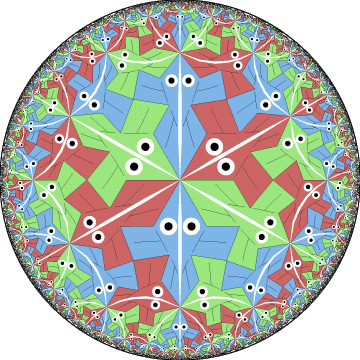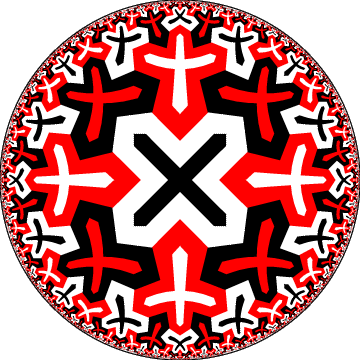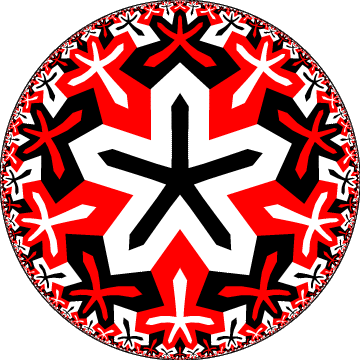## 5. Examples Based on Circle Limits I and II

M. C. Escher created four patterns using hyperbolic geometry: Circle Limit I, Circle Limit III, Circle Limit III, and Circle Limit IV. THese are shown as Catalog Numbers 429, 432, 434 (and p. 97), and 436 (and p. 98) of (Locher (1982)). We have seen renditions of Circle Limit I (Figure 3) and Circle Limit IV (Figure 1). Later we will also show renditions of Circle Limit II (Figure 7) and Circle Limit III (Figure 9). Escher's Circle Limit prints

First we consider the pattern of Figure 5 below, which shows another transformed version of the Circle Limit I pattern that is based on the {6,6} tessellation. Escher was unsatisfied with his Circle Limit I pattern (Figure 3) for three reasons:
(1) there is no "traffic flow" along the backbone lines - the fish alternate directions every two fish,
(2) the fish along a backbone line are of different colors, and
(3) the fish are angular - not nicely curved as are real fish.
Figure 5 shows a solution to the first criticism, since all the fish swim the same direction along a backbone line.Figure 5: Another transformed Circle Limit I based on {6,6}.

In examining Figure 5, we notice that three backbone lines pass through the meeting points of the fish noses. This leads to the conjecture that the pattern could be colored with three colors, one color per line of fish. Indeed this can be done as is shown by Figure 6 below. This is an answer to Escher's second criticism. Of course Escher himself successfully addressed all three criticisms with his Circle Limit III pattern (Figure 9).Figure 6: The pattern of Figure 5 colored with three colors.

Circle Limit II is the least well known of Escher's hyperbolic patterns. A rendition of Circle Limit II is shown below in Figure 7. This pattern is based on the tessellation {8,3}. There are 3-fold rotation points to the left and right at the end of each of the four cross arms. These eight 3-fold points form the vertices of a regular octagon. As with Circle Limit VI, the number of cross arms must be doubled to obtain the number of vertices of the underlying octagon, due to the bilateral symmetry of the cross arms. This pattern was undoubtedly the first repeating hyperbolic pattern with perfect 3-color symmetry.Figure 7: A rendition of Escher's Circle Limit II pattern.Figure 8: A pattern of crosses based on Circle Limit II and the {10,3} tessellation.

 INDEX NEXT